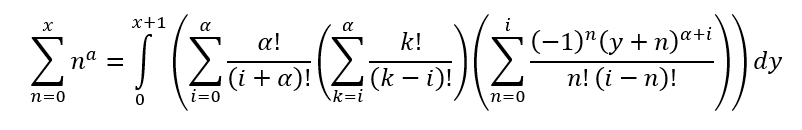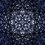# A method of evaluating sums in the general case

Ever since I learned that the sum of the first $n$ integers was $\frac { n\left( n+1 \right) }{ 2 }$, I've wondered if there was a way to generally derive an equation for the sum of ${n}^{p}$ for any positive integer $p$.

I actually did manage to find a way to do this using algebra, but it wasn't perfect. My original way was based on the fact that the telescoping sum $\sum _{ k=1 }^{ n }{ { k }^{ p }-{ \left( k-1 \right) }^{ p } } ={ n }^{ p }$. This implies that $\sum _{ n=1 }^{ x }{ \sum _{ k=1 }^{ n }{ { k }^{ p }-{ \left( k-1 \right) }^{ p } } } ={ \sum _{ n=1 }^{ x }{ { n }^{ p } } }$. There are so many ways to go from here. The idea is that the side with the double sum can be simplified using the binomial formula, and the highest order term in that representation will be $c{k}^{p-1}$ for some constant $c$. Then, you do something a bit clever and use the fact that $\sum _{ n=1 }^{ x }{ \sum _{ k=1 }^{ n }{ f\left( k \right) } } =\sum _{ n=1 }^{ x }{ \left( x-n+1 \right) f\left( n \right) }$. From here, you are left with a few summations that are doable assuming that you already know the formulas for the sums of ${n}^{q}$ for $q, and one term that requires knowledge of the sum that you are trying to find the general formula for. Luckily, this term is multiplied by a constant ($-c$ from before, in fact) that is less than $-1$, so you can do some algebra by adding it to both sides then dividing a constant out. I'm interested in the simplest form this can be brought to, as the simplest form I managed to get was

$\sum _{ n=1 }^{ x }{ { n }^{ p } } =\frac { -{ \left( -1 \right) }^{ p }x\left( x+1 \right) +p!\sum _{ n=1 }^{ x }{ \left( \sum _{ v=0 }^{ p-2 }{ \frac { { \left( -1 \right) }^{ p-v }{ n }^{ v+1 }\left[ x\left( p-v \right) +p+1 \right] }{ \left( v+1 \right) !(y-v)! } } \right) } }{ p+1 }$

which is really an absolute mess. I specifically didn't like the fact that you would need to know all previous exponent sums in order to solve this in the general case (this is to find the sum keeping $x$ as a variable).

However, I recently realized another way to find the sum that doesn't require the knowledge of the previous sums. Here it is:This is based off of the fact that, for increasing $f\left( x \right)$, $\sum _{ x=0 }^{ n }{ f\left( x \right) } \le \int _{ 0 }^{ n+1 }{ f\left( x \right)dx }$. This is obvious geometrically. Essentially, the area under the curve $f\left( x \right)$ is greater than the approximated area by left-endpoint Riemann sums using width $1$. If we let ${ a }_{ k }$ represent the $k$th derivative of some function $g\left( x \right)$, and ${a}_{k}$ is monotonic for all $k$, and ${ a }_{ k }=0$ eventually (for all $x$), then the simple observation I mentioned earlier in this paragraph can be used to rewrite $\sum _{ x=a }^{ b }{ g\left( x \right) }$ as something inside of an integral. The bounds on the summation only directly influence the bounds on the integral, so this method is useful for generalizing sums.

I'll write out either derivation if there is any interest in seeing them, otherwise I'll leave any derivation and further thoughts/application to you.Note by Austin Antonacci
3 years, 9 months ago

This discussion board is a place to discuss our Daily Challenges and the math and science related to those challenges. Explanations are more than just a solution — they should explain the steps and thinking strategies that you used to obtain the solution. Comments should further the discussion of math and science.

When posting on Brilliant:

• Use the emojis to react to an explanation, whether you're congratulating a job well done , or just really confused .
• Ask specific questions about the challenge or the steps in somebody's explanation. Well-posed questions can add a lot to the discussion, but posting "I don't understand!" doesn't help anyone.
• Try to contribute something new to the discussion, whether it is an extension, generalization or other idea related to the challenge.

MarkdownAppears as
*italics* or _italics_ italics
**bold** or __bold__ bold
- bulleted- list
• bulleted
• list
1. numbered2. list
1. numbered
2. list
Note: you must add a full line of space before and after lists for them to show up correctly
paragraph 1paragraph 2

paragraph 1

paragraph 2

[example link](https://brilliant.org)example link
> This is a quote
This is a quote
    # I indented these lines
# 4 spaces, and now they show
# up as a code block.

print "hello world"
# I indented these lines
# 4 spaces, and now they show
# up as a code block.

print "hello world"
MathAppears as
Remember to wrap math in $$ ... $$ or $ ... $ to ensure proper formatting.
2 \times 3 $2 \times 3$
2^{34} $2^{34}$
a_{i-1} $a_{i-1}$
\frac{2}{3} $\frac{2}{3}$
\sqrt{2} $\sqrt{2}$
\sum_{i=1}^3 $\sum_{i=1}^3$
\sin \theta $\sin \theta$
\boxed{123} $\boxed{123}$

Sort by:

- 3 years, 9 months ago

Thanks! I'm having fun just messing around with the sum, but I'll look into that.

- 3 years, 9 months ago